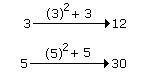# Verbal Reasoning - Analogy - Discussion

Discussion Forum : Analogy - Analogy 3 (Q.No. 5)
Directions to Solve
In each of the following questions find out the alternative which will replace the question mark.

5.
3 : 12 :: 5 : ?
25
35
30
15
Explanation:Discussion:
17 comments Page 1 of 2.

Rajesh Shahi said:   1 year ago
11:35::17:?

Mayur said:   2 years ago
N*(N+1):
3*(3+1) = 12.
5*(5+1) = 30.

Syed abbaas Rixvii said:   2 years ago
Agree with you, Thanks @Sayali Borse.

Alankrita said:   3 years ago
Simple explanation is;

3 * 4 = 12.
In the second proportion;
The First digit is 5 and the next digit is 6.
So, 5 * 6=30.
Now,
3:3 * 4 :: 5 : 5 : 6.
3:12::5::30.

Sayali borse said:   3 years ago
The best solution to understand this is:
What comes after 3 is 4.
So 3*4 = 12.

What's come after 5 is 6.
5*6 = 30.

Abhishek Dutta said:   4 years ago

1*2+1 = 3,
3*3+3 = 12.

And;
4*1+1 = 5,
6*2+3 = 15.

Suhani kushwaha said:   6 years ago
3*4=12 like this 5*4=20.

Brijesh said:   6 years ago
3:15::11 :?

Solve this.

Pearl said:   6 years ago
3 and 5 are consecutive prime numbers so,

3 * 4 = 12 and 5 * 4 = 20 so 20 also can be the answer.

Bhargav said:   6 years ago
3 *4 = 12
same as 5 * 4 = 20.